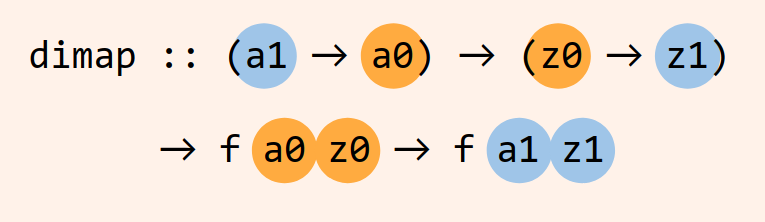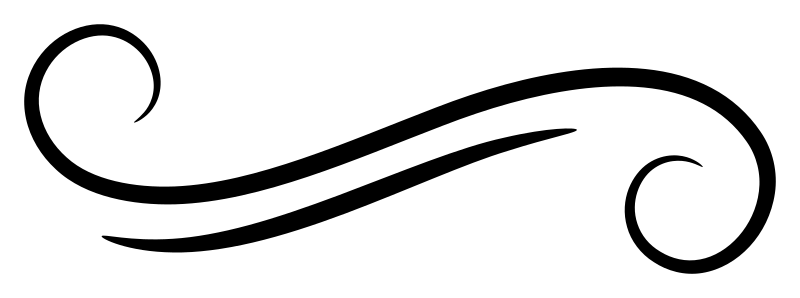# Understanding profunctors

Profunctors are bifunctors that are contravariant in their first type argument and covariant in their second one. Make sure that you understand contravariance first. Then we just need to talk about bifunctors, and finally we will get to profunctors.

## `Bifunctor`

`Bifunctor`, which is available in `base`, is a lot like `Functor`. It offers a nice solution for those times when you don’t want to ignore the leftmost type argument of a binary type constructor, such as `Either` or `(,)`. Its core operation, `bimap`, closely resembles `fmap`, except it lifts two functions into the new context, allowing you to apply one or both.

``````class Bifunctor (f :: * -> * -> *) where
bimap :: (a0 -> z0)
-> (a1 -> z1)
-> f a0 a1
-> f z0 z1``````

Ye gods that’s a lot of variables! Let’s clean that up a bit. We’ll be talking about the `bimap` for `Either` and tuples so let’s go ahead and see what those look like:Each `@` symbol in these examples is a visible type application, using the `TypeApplications` GHC language extension.

``````bimap @Either :: (a0 -> z0)
-> (a1 -> z1)
-> Either a0 a1
-> Either z0 z1``````
``````bimap @(,) :: (a0 -> z0)
-> (a1 -> z1)
-> (a0, a1)
-> (z0, z1)``````

`bimap` takes two unary functions as arguments along with a value, such as `(1, 3)` or `Left 5`, and applies whichever function it can – both if it can! We’ll partially apply `bimap` here so that we can reuse it:

``````greet :: Bifunctor p => p String String
-> p String String
greet = bimap ("hello " ++) ("goodbye " ++)``````

That `p` is going to be something like `Either` or `(,)`, taking two type arguments, although in this case we already know both those type arguments have to be strings. Let’s try it out.

``````λ> greet (Left "Julie")
Left "hello Julie"

λ> greet (Right "March")
Right "goodbye March"``````

We can use the function on `Either` values, even though only one “side” is present at the value level at a time. We can also use it on a two-tuple and use both functions at once:

``````λ> greet ("Julie", "to all that")
("hello Julie","goodbye to all that")``````

So, `bimap` is `fmap` but for binary type constructors where you want the ability to lift two functions at once.

## Profunctor

Profunctors are bifunctors that are contravariant in the first argument and covariant in the second one. While people do incredibly magical looking things with profunctors, if you’ve understood `fmap`, `contramap`, and `bimap`, then you’re ready for `dimap`.

(That’s `di` as in “dioxide”; `bi` was already in use for `bimap`, so we had to switch from Latin to Greek.)

 `Bifunctor` `bimap` “bi” as in bicycle “2” in Latin `Profunctor` `dimap` “di” as in dioxide “2” in Greek

The core operation of the `Profunctor` class is `dimap` – get ready for some type variable soup.

``````class Profunctor (f :: * -> * -> *) where
dimap :: (a1 -> a0)
-> (z0 -> z1)
-> f a0 z0
-> f a1 z1``````

We can start by looking at where it differs from the `Bifunctor` definition: We have renamed all of the type variables for the moment, just to highlight this particular comparison.

``````bimap :: (a -> b) -> (c -> d) -> f a c -> f b d
--        ^^^^^^
dimap :: (b -> a) -> (c -> d) -> f a c -> f b d
--        ^^^^^^``````

Earlier we saw what `bimap` looks like with `Either` and tuple types, but we cannot also implement `dimap` for these types. That’s because of the contravariance in the first argument; as we saw with `Contravariant`, basically everything in Haskell that are contravariant functors are function types.

Informally, what we’re going to have is a bifunctor that acts like `fmap` on the `z` of `f a z` and like `contramap` on the `a` of `f a z`. It’s worth pointing out here that the `Profunctor` class also has methods called `lmap` and `rmap` (for left and right map, respectively), and their implementations for the function type are `flip (.)` and `(.)`. There’s a lot of function composition going on under the hood here.

## The `(->)` profunctor

Since we’ve been talking about about functors of functions, we’re going to continue to do so as that is the simplest profunctor example we could start with.

What `dimap f g` does is take `h` and squeeze it in between `f` and `g`.

``dimap f g h = g . h . f``

Or, to put it another way, `dimap f g` starts with `f a0 z0` and

1. Extends it on the input side by applying `f :: a1 -> a0` to change the “argument” type variable from `a0` to `a1` (this is the contravariant part).
2. Extends it on the output side by applying `g :: z0 -> z1` to change the “result” type variable from `z0` to `z1` (this is the covariant part).

Thus giving a result of `f a1 z1`.## Words and phrases

We’ll take a contrived but uncomplicated example and step very carefully through the flow of types. We will annotate the types as best we can to try to make it more clear.

``````type Word   = String
type Phrase = String

capWord :: Word -> Word
capWord [] = []
toUpper head : fmap toLower tail

capPhrase :: Phrase -> Phrase
capPhrase =
dimap words unwords (fmap capWord)``````

This `dimap`, since we’re working with the function profunctor, takes three functions as input. They are:

``````words        :: Phrase -> [Word]
unwords      :: [Word] -> Phrase
fmap capWord :: [Word] -> [Word]``````
``````dimap
:: (a1 -> a0) -- f - words        :: Phrase -> [Word]
-> (z0 -> z1) -- g - unwords      :: [Word] -> Phrase
-> p a0 z0    -- h - fmap capWord :: [Word] -> [Word]
-> p a1 z1    --                     Phrase -> Phrase``````
1. The `Phrase` argument `a1` gets passed to the `words` function first, converting it to type `a0`, `[Word]`.
2. Where does the `a0` go? To function `h`, the next in our series of composed functions, which in this case is `fmap capWord`, producing a `z0` output, which is still, for this example, a `[Word]`.
3. That `z0` output gets handed off to `unwords` next, the last link in the chain of composition, and becomes `z1`, which is a `Phrase` again.
``````λ> capitalize "Julie loves DONUTS"
"Julie Loves Donuts"``````

You can pull out the `words . unwords` not-quite-isomorphism by partially applying `dimap`, in case you have other ways in which you’d like to alter phrases as lists of words:

``````withPhraseAsWords :: Profunctor f
=> f [Word] [Word] -> f Phrase Phrase
withPhraseAsWords = dimap words unwords

capPhrase :: Phrase -> Phrase
capPhrase = withPhraseAsWords (fmap capWord)

takeTwoWords :: Phrase -> Phrase
takeTwoWords = withPhraseAsWords (take 2)``````
``````λ> capPhrase "one two three"
"One Two Three"

λ> takeTwoWords "one two three"
"one two"
it :: Phrase``````Join Type Classes for courses and projects to get you started and make you an expert in FP with Haskell.